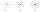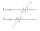# Ratio + line segment - math problems

On solving problems and tasks with proportionally, we recommend hint rule of three. Rule of three (proportionality) help solve examples of direct and inverse proportionality. Three members make possible to calculate the fourth - unknown member.

#### Number of problems found: 11

• Similarity coefficientIn the triangle TMA the length of the sides is t = 5cm, m = 3.5cm, a = 6.2cm. Another similar triangle has side lengths of 6.65 cm, 11.78 cm, 9.5 cm. Determine the similarity coefficient of these triangles and assign similar sides to each other.
• Divide in ratioLine segment AB 12 cm long divide in a ratio of 5: 3. How long are the individual parts?
• Line segmentThe 4 cm long line segment is enlarged in the ratio of 5/2. How many centimeters will measure the new line segment?
• Trapezoid - intersection of diagonalsIn the ABCD trapezoid is AB = 8 cm long, trapezium height 6 cm, and distance of diagonals intersection from AB is 4 cm. Calculate trapezoid area.
• Points on line segmentPoints P & Q belong to segment AB. If AB=a, AP = 2PQ = 2QB, find the distance: between point A and the midpoint of the segment QB.
• Line segmentCut a line segment of 15 cm into two line segments so that their lengths are in ratio 2:1. What length will each have?
• Sides od triangleSides of the triangle ABC has length 4 cm, 5 cm and 7 cm. Construct triangle A'B'C' that are similar to triangle ABC which has a circumference of 12 cm.
• Map 2At what scale is made map if the distance 8.2 km corresponds on the map segment 5 cm long?
• Line segmentFor the line segment whose endpoints are L[-1, 13] and M[18, 2], find the x and y value for the point located 4 over 7 the distance from L to M.
• SlopeCalculate the slope of a line that intersects points (-84,41) and (-76,-32).
• Line segmentLine segment AB is 8 cm long. Divide it in a ratio of 2: 3.

We apologize, but in this category are not a lot of examples.
Do you have an interesting mathematical word problem that you can't solve it? Submit a math problem, and we can try to solve it.

We will send a solution to your e-mail address. Solved examples are also published here. Please enter the e-mail correctly and check whether you don't have a full mailbox.

Please do not submit problems from current active competitions such as Mathematical Olympiad, correspondence seminars etc...

Check out our ratio calculator.